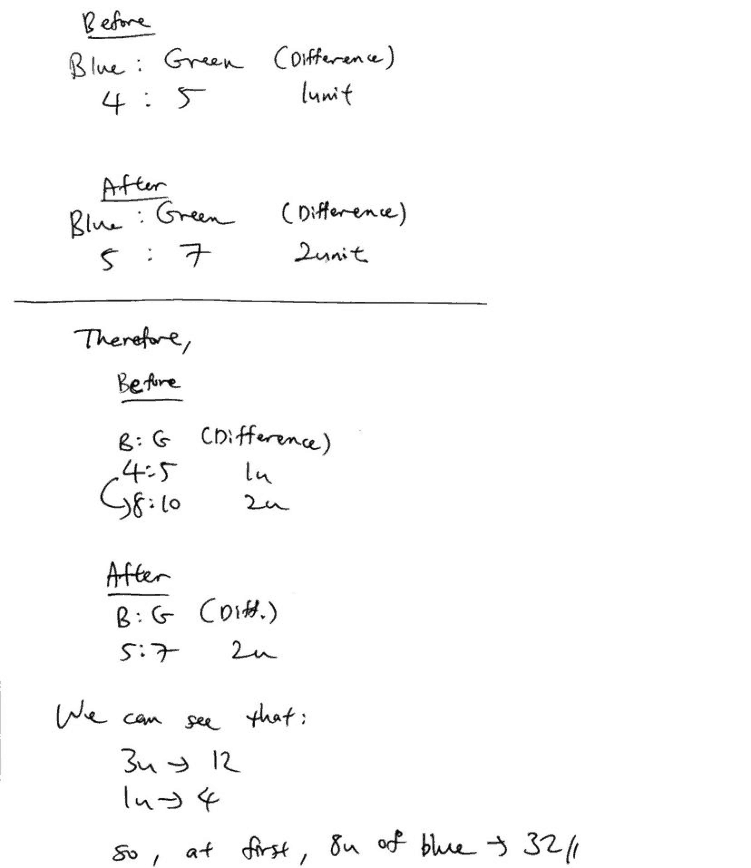# Question

 Blue Green Before 4 units 5 units Change -12 -12 After 5 parts 7 parts

Multiply Blue’s column by 7 and Green’s column by 5 to get equal parts:

 Blue Green 28 units 25 units -84 -60 35 parts 35 parts

Comparing the two columns:

28 units – 84 = 25 units – 60
3 units = 24
1 unit = 8

Blue balloons at first = 4 units
= 4 × 8
= 32

0 Replies 0 Likes ✔Accepted AnswerThis is a problem sum dealing with a concept called Constant Difference.

This means that the difference between the blue and green balloons should remain the same  for the ‘before’ situation and the ‘after’ situation. This is because an EQUAL amount of blue and green balloons were given away. This causes the difference between the different colours to remain the same. Likewise, the difference will remain the same if Bob receives an EQUAL amount of blue and green balloons from someone else.

Looking at the solution provided, we can see that the difference in the ‘before’ is 1 unit while the difference in the ‘after’ is 2 units. Remember, that the difference should remain the same as this is a Constant Difference question. So, we make it the same by doing some manipulation to the ratio of ‘4 : 5’, and change it into its complex form ‘8 : 10″, so that now we can observe that the difference for the ‘before’ is 2 units, just like the ‘after’.

By observation, we can compare the two ratio we have now obtained, ‘8 : 10’ and ‘5 : 7’. We can see that each colour has its units decreased by 3 units. This shows us that these 3 units corresponds to our value of 12 balloons of each colour. So, 3 units represents 12 balloons, 1 unit represents 4 balloons.

To answer the question, there were 8 units of Blue balloons at first, so that makes it 32.

We provide coaching for small, focus groups of students at Future Achievers Hub at Tampines Junction.

Do check out:

www.futureachievers.com.sg

or call us at +65 83325521,